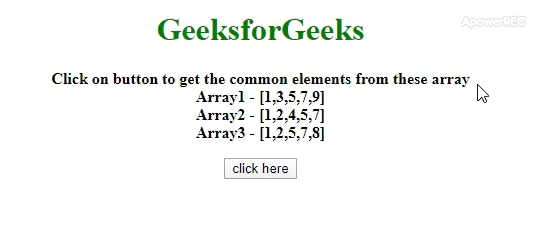# Find the common elements of more than two JavaScript arrays ?

Given an HTML document having multiple arrays with some elements and the task is to get the common elements from arrays with the help of JavaScript. There are two approaches that are discussed below.

Approach 1: First get the arrays in 2-dimensional format then take the first array by shift() method and then filter out the elements from the first array which are common in all by using filter() method. The elements which are common in all arrays can be checked by every() method and if the element of first array matches with all elements then that element is returned.

• Example:

 ` ` `<``html``> ` ` `  `<``head``> ` `    ``<``title``> ` `        ``Finding matches between more than ` `        ``2 JavaScript arrays ` `    `` ` `    ``<``style``> ` `        ``body { ` `            ``text-align:center; ` `        ``} ` `        ``h1 { ` `            ``color: green; ` `        ``} ` `        ``#geeks { ` `            ``font-size: 16px;  ` `            ``font-weight: bold; ` `        ``} ` `         `  `        ``#gfg { ` `            ``color: green;  ` `            ``font-size: 18px;  ` `            ``font-weight: bold; ` `        ``} ` `    `` ` ` ` ` `  `<``body` `style``=``""``> ` `    ``<``h1` `>  ` `            ``GeeksforGeeks  ` `        `` ` `    ``<``p` `id``=``"geeks"``> ` `    `` ` `    ``<``button` `onClick``=``"GFG_Fun()"``> ` `        ``click here ` `    `` ` `    ``<``p` `id``=``"gfg"` `> ` `    `` ` `    ``<``script``> ` `        ``var up = document.getElementById('geeks'); ` `        ``var down = document.getElementById('gfg'); ` `        ``var arr1 = [1, 3, 5, 7, 9]; ` `        ``var arr2 = [1, 2, 4, 5, 7]; ` `        ``var arr3 = [1, 2, 5, 7, 8]; ` `        ``var arr = [arr1, arr2, arr3]; ` `        ``up.innerHTML = ` `            ``"Click on button to get the common elements from these"+ ` `            ``" array<``br``>Array1 - [" + arr1 + "]<``br``>Array2 -"+ ` `            ``" [" + arr2 + "]<``br``>Array3 - [" + arr3 + "]"; ` ` `  `        ``function GFG_Fun() { ` `            ``arr4 = arr.slice(); ` `            ``down.innerHTML = arr4.shift().filter(function(v) { ` `                ``return arr4.every(function(a) { ` `                    ``return a.indexOf(v) !== -1; ` `                ``}); ` `            ``}); ` `        ``} ` `    `` ` ` ` ` `  ` `

• Output:Approach 2: First get the arrays in 2-dimensional format then use the reduce() method to get the access to all arrays one by one. variable(p) contains each array one by one and returns only elements of the array which are in variable(c) array by using filter() method. At the end variable(c) contains the array of common elements.

• Example:

 ` ` `<``html``> ` ` `  `<``head``> ` `    ``<``title``> ` `        ``Finding matches between more than ` `        ``2 JavaScript arrays ` `    `` ` `    ``<``style``> ` `        ``body { ` `            ``text-align:center; ` `        ``} ` `        ``h1 { ` `            ``color: green; ` `        ``} ` `        ``#geeks { ` `            ``font-size: 16px;  ` `            ``font-weight: bold; ` `        ``} ` `         `  `        ``#gfg { ` `            ``color: green;  ` `            ``font-size: 18px;  ` `            ``font-weight: bold; ` `        ``} ` `    `` ` ` ` ` `  `<``body` `style``=``""``> ` `    ``<``h1` `>  ` `     ``GeeksforGeeks  ` `    `` ` `    ``<``p` `id``=``"geeks"``> ` `    `` ` `    ``<``button` `onClick``=``"GFG_Fun()"``> ` `        ``click here ` `    `` ` `    ``<``p` `id``=``"gfg"` `> ` `    `` ` `    ``<``script``> ` `        ``var up = document.getElementById('geeks'); ` `        ``var down = document.getElementById('gfg'); ` `        ``var arr1 = [1, 3, 5, 7, 9]; ` `        ``var arr2 = [1, 2, 4, 5, 7]; ` `        ``var arr3 = [1, 2, 5, 7, 8]; ` `        ``var arr = [arr1, arr2, arr3]; ` `        ``up.innerHTML = ` `            ``"Click on button to get the common elements from these"+ ` `            ``" array<``br``>Array1 - [" + arr1 + "]<``br``>Array2 -"+ ` `            ``" [" + arr2 + "]<``br``>Array3 - [" + arr3 + "]"; ` ` `  `        ``function GFG_Fun() { ` `            ``down.innerHTML = arr.reduce((p, c) => ` `            ``p.filter(e => c.includes(e))); ` `        ``} ` `    `` ` ` ` ` `  ` `

• Output:My Personal Notes arrow_drop_upCheck out this Author's contributed articles.

If you like GeeksforGeeks and would like to contribute, you can also write an article using contribute.geeksforgeeks.org or mail your article to contribute@geeksforgeeks.org. See your article appearing on the GeeksforGeeks main page and help other Geeks.

Please Improve this article if you find anything incorrect by clicking on the "Improve Article" button below.

Practice Tags :

Be the First to upvote.

Please write to us at contribute@geeksforgeeks.org to report any issue with the above content.